Next: Luminosity Integration Up: Solving Equation of Motion Previous: Equation of motion under

### Equation of motion under PUSH command

Solving the equation of motion in PUSH command is much more complicated because of the possible presense of the beam field. The equation of motion is in general written in the form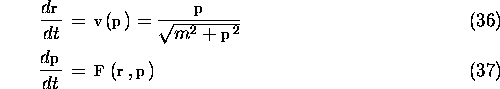The force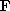includes the beam field and the external field. The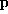dependence ofcomes from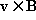although very weak in the case of the beam field.

Given the initial variables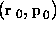, a simple approximation after the time interval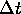is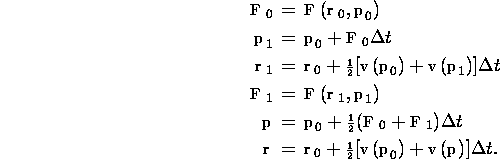The error ofby these formulas is estimated by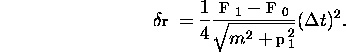If this is not small enough, divide the intervalby an integer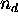. Note that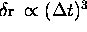because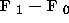is proportional to. The total error, after multiplied by the number of intervals, is proportional to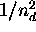.

However, the above prescription is not really enough when there are extremely low energy particles (e.g., those from incoherent pair creation). It often happens thatso determined bocomes over several hundreds. In such a case the above error estimation may not be accurate at all.

Whenis too large, CAIN tries the fourth-order Runge-Kutta integration. Starting from the whole interval, it is divided by 2 at each step until the difference becomes small enough. This method is a little better than the simple formulas above but is still time consuming. So, the users should be aware that incoherent pair creation is expensive.

Toshiaki Tauchi
Thu Dec 3 17:27:26 JST 1998